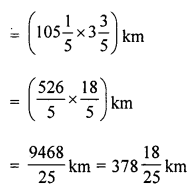## ML Aggarwal Class 6 Solutions for ICSE Maths Chapter 8 Ratio and Proportion Ex 8.5

Question 1.
The speed of a car is $$105 \frac{1}{5}$$ km/h, find the distance covered by it in $$3 \frac{3}{5}$$ hours.
Solution:
Speed of a car = $$105 \frac{1}{5}$$ km/h
Distance covered by car in = $$3 \frac{3}{5}$$ hours
= Speed × TimeQuestion 2.
If the speed of a car is 50.4 km/h, find the distance covered in 3.6 hours.
Solution:
Speed of a car = 50.4 km/h
∴ Distance covered in 3.6 hours
= Speed × Time
= (50.4 × 3.6) km/h
= 181.44 km

Question 3.
If a car covers a distance of 201.25 km in 3.5 hours, find the speed of the car.
Solution:
Distance covered by the car = 201.25 km
and time consumed by car = 3.5 hours
∴ The speed of car = $$\frac{\text { Distance }}{\text { Time }}$$
= $$\frac{201.25}{3.5}$$ km/h = 57.5 km/h